## Tuesday, July 12, 2011

### MathJax in Blogger (II)

The new awesome way to include math in a website is MathJax. Until recently, one had to install MathJax in their own server in order to include math in their webpages. That changed a few weeks ago; you can now specify in your html code that the required script be loaded from MathJax's website.

To get MathJax to work in Blogger, just go to your Blogger account, click "Design" (top right of the page), and then "Edit HTML". After the first <head> you see, paste
<script src='http://cdn.mathjax.org/mathjax/latest/MathJax.js' type='text/javascript'>
MathJax.Hub.Config({
HTML: ["input/TeX","output/HTML-CSS"],
TeX: { extensions: ["AMSmath.js","AMSsymbols.js"],
equationNumbers: { autoNumber: "AMS" } },
extensions: ["tex2jax.js"],
jax: ["input/TeX","output/HTML-CSS"],
tex2jax: { inlineMath: [ ['$','$'], ["\$","\$"] ],
displayMath: [ ['$$','$$'], ["\$","\$"] ],
processEscapes: true },
"HTML-CSS": { availableFonts: ["TeX"],
linebreaks: { automatic: true } }
});
</script>

You're done! You can now use the usual $...$ or $...$ for inline equations, and $$...$$ or
$...$ for equations that are centered in their own line.

Time for an example:
$\cos^2\theta-\sin^2\theta=\cos 2\theta.$ The above script loads AMSmath and AMSsymbols so that the macros defined in these popular packages are available for use in your blog.

UPDATE: Equations can now be numbered and referred to. For example, in Newtonian gravity we have
\begin{equation}\label{eq:gravt}
F=G\frac{mM}{r^2}.
\end{equation}Also,
\begin{equation}\label{eq:gravo}
F=mg,
\end{equation}and so equations (\ref{eq:gravt}) and (\ref{eq:gravo}) lead to$g=G\frac{M}{r^2},$assuming the equivalence of inertial and gravitational mass.

1.Awesome post $\cos^2\theta-\sin^2\theta=\cos 2\theta$

1.no funtiona en celulares es decir todas las formulas de aqui se pueden ver en computadoras pero no en celulares una pena

2.Para que funcione en celulares hay que personalizar la plantilla para móviles:

loguear en la cuenta Bloogger e ir al blog, click en Plantilla->click en la ruedita de plantilla para móvil->seleccionar "Sí, mostrar la plantilla para móviles...." ->
En el menú desplegable "Elegir plantilla para móviles" seleccionar "Personalizado". Guardar y listo.

Para ver la versión para móvil desde una computadora hay que agregar "?m=1" al final de la url. Por ejemplo, la versión para móvil de esta página es http://irrep.blogspot.com/2011/07/mathjax-in-blogger-ii.html?m=1

Es verdad lo que dices, no se ven la fórmulas porque este blog no tiene plantilla para móvil personalizada.

Si miras la versión para móvil de mi blog verás que todas las fórmulas se ven perfectamente:

2.Thanks for the tips!

3.Great post. Anything to make font bigger

4.@SeerSucker The font should scale with the font you use in your blog.

5.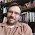Right click the equation and you can set the zoom factor and zoom trigger in the settings option.

6.I've tried that.Wonderful.$x^n+y^n=z^n$

7.I successfully used your JS in Blogger. Thanks for that.

One thing that seems to be missing is equation numbering. Any mods to your script to enable that?

1.8.Nice! Now applied to my blog.

FYI: I noticed that, since the numbering is applied globally then, with numbered eqns in say 2 separate blog posts, the first eqn in the older post will not be (1) but some higher number, e.g., (6), depending on how many numbered eqns precede it in the newer post.

In LaTeX, the eqn counter can be reset as desired. Is there a similar feature in MathJax?

9.Thank you.Let me try
\begin{equation}
x^n+y^n=z^n
\end{equation}

10.\begin{eqnarray}
\begin{array}{rcl}
\alpha\beta & = & {1\over
2}\left(\alpha\otimes\beta+\beta\otimes\alpha\right),\\
\\
\alpha\wedge\beta & = & {1\over
2}\left(\alpha\otimes\beta-\beta\otimes\alpha\right).
\end{array}
\end{eqnarray}

1.This comment has been removed by the author.

11.i followed your steps but im unable to get it working on my blog.. where am i going wrong? can you guide me a bit? Thanks

12.This comment has been removed by the author.

13.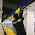Thanks mate, it is just simply what I need. To write $3x+6y=8$ in my blog. :)

14.Do the formulas work in Preview mode? In my case, they don't.

Thanks.

1.In my case the formulas work in Preview mode.

15.How is anyone getting this to work? I just created a Blogger account. I see no "Design" option anywhere, and I certainly see no way to edit the raw HTML header of my blog.

I had a mathematics blog on a Joomla site a while back, where I had written a Joomla plugin to render LaTeX. That site is gone. I'd like to migrate that blog to Blogger, but not if I can't get MathJax working.

1.Ah, I almost got it. On my dashboard, left column, I click on Template. Under the thumbnail shown as "Live on Blog" I click "Edit HTML" and insert the javascript into the header.

The problem is, it still isn't working.

2.Aha!

Andy posted this in 2011, and apparently things have changed, according to what I have found in the Google support forum.

The new dynamic view blogger themes make integrating MathJax a bit harder now.

To get MathJax to work in the modern Blogger, you have to insert the following Typeset() method call at the end of each post:

<script type="text/javascript">MathJax.Hub.Queue(["Typeset",MathJax.Hub]);</script>

See http://docs.mathjax.org/en/latest/typeset.html

There may be a place to insert this call near the end of the template, but I haven't found any place where this works. It works only if you insert it at the end of each blog post.

Unfortunately, this is kind of a kludge. And won't allow for math formulas in comments.

3.i put it on the end of the html code of a post and it didnt work

4.16.Well updating again regarding blogger. In my blogger is working fine just the numbering option that still not working....

1.Updating it's working perfectly as long is you don't change anything in style, font syze, font type etc.... Any change on those things will include extra html code and mess-up with MathJax.

2.Again... heheh just adding works perfectly with simple view.

17.AWESOME MAAAAAN

18.Work perfectly!!

19.Thanks! I was hoping for a simple explanation and you delivered!

20.21.\begin{equation}
\frac{1+x+x^2}{1+y+y^2}
\end{equation}

22.$a^2 + b^2 = c^2$

1.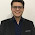$\sin(\Theta)\cdot \cos (\Omega )$

23.Good work

tsg
mbaapti.blogspot.com

24.25.Nice! thanks for sharing.

26.Works great, thanks for sharing.

27.$\mathbf{V}_1 \times \mathbf{V}_2 = \begin{vmatrix} \mathbf{i} & \mathbf{j} & \mathbf{k} \\ \frac{\partial X}{\partial u} & \frac{\partial Y}{\partial u} & 0 \\ \frac{\partial X}{\partial v} & \frac{\partial Y}{\partial v} & 0 \end{vmatrix}$

28.This comment has been removed by the author.

29.this not work for cell , esta aplicacion no se puede ver en celulares

30.$\sum_{k=0}^\infty x^k = \frac{1}{1-x}$

31.$x^2 + y^2 = 1$

32.$x^n + y^n = z^n$

33.\begin{equation} \frac{1+\sqrt{5}}{2} \end{equation}

34.$E = mc^2$

1.it doesnt work on my blog

2.me neither...you ever get it to work?

35.this worked great! Thanks for posting

36.awesome... thank you for this post

37.Can I try as well: $x^2+y^2=r^2$.

38.You're a legend. Thank you so much!!!

39.$\mathbf{V}_1 \times \mathbf{V}_2 = \begin{vmatrix} \mathbf{i} & \mathbf{j} & \mathbf{k} \\ \frac{\partial X}{\partial u} & \frac{\partial Y}{\partial u} & 0 \\ \frac{\partial X}{\partial v} & \frac{\partial Y}{\partial v} & 0 \end{vmatrix}$

I can't for the life of me get this to work. I've tried like 40 different things too....sigh.

40.This complen in my blog

http://www.idmathcirebon.com

41.This comment has been removed by the author.

42.Testing
\lim_{x \to \infty} \exp(-x) = 0

1.It doesn't seem to work
\theta + \cos^2 (2\theta)

2.$\lim_{x \to \infty} \exp(-x) = 0$

43.\cos^2\theta

1.What am I doing wrong here?
\frac{1+\sqrt 5} 5

2.You have to enclose the math in dollar signs for equations to be displayed (see article).

3.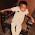$$\cos^2\theta$$

$$\frac{1+\sqrt 5} 5$$

44.$cos^2 x - sin^2x = Cos2x ; 1+ sqrt5 / 2...$

45.$(1 + sqrt 5 )/ 2 ...$

46.$$\tan \alpha = \frac{\sin \alpha}{\cos \alpha}$$

47.Most of the universities and universities and universities create it compulsory for candidates to publish articles on a pre-defined subject. This helps them in the choice oftraveling for students potential students for entrance into their educational institution.

48.great post - very useful!

49.50.Website templates are a great way of creating a website without having to spend weeks designing and developing web pages.
Please visit us: html and css

51.This comment has been removed by the author.

52.When $a \ne 0$, there are two solutions to $ax^2 + bx + c = 0$ and they are
$$x = {-b \pm \sqrt{b^2-4ac} \over 2a}.$$

53.This comment has been removed by the author.

54.This comment has been removed by the author.

55.This comment has been removed by the author.

56.57.$\cos^2\theta-\sin^2\theta=\cos 2\theta$

58.This comment has been removed by the author.

59.Thanks, so munch. Maybe I can remind the guys who noticed this reply: If you wanna try, remember do not copy a Latex from web with format, you can just paste as plain text then you will see the formula in preview page. If you know this, ignore me.

60.This comment has been removed by the author.

61.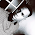62.check this equation $ax + bx =c$

63.This comment has been removed by the author.

64.Super post..its working for me

65.Please someone help me on resolving the issue for my blog, here AsciiMath (Like frac, sqrt etc) is not showing properly.
https://www.studypivot.com/logarithm-rules-and-examples/
I used above code but it won't worked, so I used the following code.

src="http://cdn.mathjax.org/mathjax/latest/MathJax.js">
MathJax.Hub.Config({
extensions: ["tex2jax.js","TeX/AMSmath.js","TeX/AMSsymbols.js"],
jax: ["input/TeX", "output/HTML-CSS"],
tex2jax: {
inlineMath: [ ['$','$'], ["\$","\$"] ],
displayMath: [ ['$$','$$'], ["\$","\$"] ],
},
"HTML-CSS": { availableFonts: ["TeX"] }
});

66.Can the sections and subsections be shown well? i could only show the fonts and equations.

67.testing basic stuff vector $\overrightarrow{v}$ , boolean $\overline{A}$ , matrix ${\begin{array}{cc}A&B&C\\ D&E&F\\ G&H&I\end{array}}$ , the n-th root $\sqrt[n\ \ \ ]{x}$ , closes ${\left({\frac{A-B}{A+B}}\right)}^{\frac{A-B}{A+B}}$ , sum $\diplaystyle{\sum_{i=1}^n{i}}$ , lim $\diplaystyle{\lim_{i→1\\ i\in\mathbb{R}}{\frac1{1-i}}}$

68.keyofix : , sum $\displaystyle{\sum_{i=1}^n{i}}$ , lim $\displaystyle{\lim_{i→1\\ i\in\mathbb{R}}{\frac1{1-i}}}$

69.70.This comment has been removed by the author.

71.72.When $a \ne 0$, there are two solutions to $ax^2 + bx + c = 0$ and they are
$x = {-b \pm \sqrt{b^2-4ac} \over 2a}.$